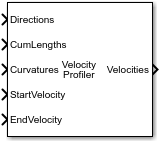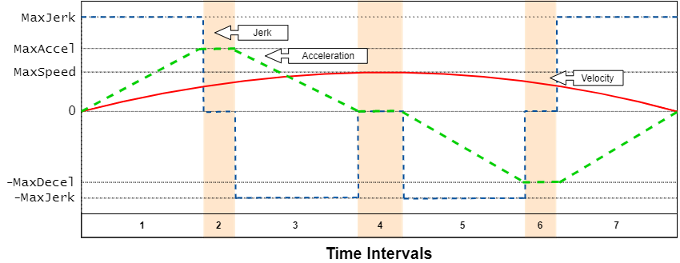# Velocity Profiler

Generate velocity profile of vehicle path given kinematic constraints

Since R2019b

•Libraries:
Automated Driving Toolbox

## Description

The Velocity Profiler block generates a velocity profile of a driving path that satisfies this set of specified kinematic constraints:

• The maximum allowable speed of the vehicle

• The maximum longitudinal acceleration and deceleration of the vehicle

• The maximum longitudinal jerk of the vehicle

• The maximum lateral acceleration of the vehicle

Specify the cumulative lengths along the path and the driving directions and curvatures at each point along the path. You can obtain these values from the output of a Path Smoother Spline block. Also specify the longitudinal velocity of the vehicle at the start and end of the path.

Use the generated velocity profile as the input reference velocities of a longitudinal controller, as shown in the Automated Parking Valet in Simulink example.

## Ports

### Input

expand all

Driving directions of the vehicle along the length of the path, specified as an M-by-1 vector of 1s (forward motion) and –1s (reverse motion). Each vector element represents the driving direction of the vehicle at the corresponding cumulative path length specified by the CumLengths input port. M is the number of driving directions and must be equal to the lengths of the CumLengths and Curvatures inputs.

You can obtain Directions from the output of a Path Smoother Spline block.

Cumulative path lengths, in meters, specified as an M-by-1 vector of monotonically increasing real-valued elements. Each vector element represents a point along the path. M is the number of cumulative path lengths and must be equal to the lengths of the Directions and Curvatures inputs.

You can obtain CumLengths from the output of a Path Smoother Spline block.

Signed path curvatures along the length of the path, in radians per meter, specified as an M-by-1 real-valued vector. Each vector element represents the curvature of the path at the corresponding cumulative path length specified by the CumLengths input port. M is the number of curvatures and must be equal to the lengths of the Directions and CumLengths inputs.

You can obtain Curvatures from the output of a Path Smoother Spline block.

Longitudinal velocity of the vehicle at the start of the path, in meters per second, specified as a real scalar.

Longitudinal velocity of the vehicle at the end of the path, in meters per second, specified as a real scalar.

### Output

expand all

Velocity profile along the length of the path, in meters per second, returned as an M-by-1 real-valued column vector. Each vector element represents a reference longitudinal velocity for the vehicle at the corresponding cumulative path length specified by the CumLengths input port. M is the number of velocities and is equal to the length of CumLengths.

The output velocity values satisfy the speed, acceleration, and jerk constraints specified in the parameters of the Velocity Profiler block. You can use this output as the reference velocity for a vehicle controller.

Velocities is a variable-size output with the limitations described in Variable-Size Signal Limitations (Simulink).

Vehicle times of arrival for the velocity profile specified in Velocities, returned as an M-by-1 real-valued vector. M is the number of vehicle times of arrival and is equal to the length of Velocities. Units are in seconds.

Each vector element represents the time that a vehicle traveling at velocity v arrives at cumulative path length p, where:

• v is the corresponding velocity returned by the Velocities output port.

• p is the corresponding cumulative path length specified by the CumLengths input port.

Use Times to visualize the velocity profile over time, as shown in the Velocity Profile of Straight Path and Velocity Profile of Path with Curve and Direction Change examples.

Times is a variable-size output with the limitations described in Variable-Size Signal Limitations (Simulink).

#### Dependencies

To enable this port, select the Show Times output port parameter.

## Parameters

expand all

Maximum longitudinal acceleration of the vehicle, in meters per second squared, specified as a positive real scalar.

When developing a longitudinal controller, this parameter must be equal to the corresponding parameter in the Longitudinal Controller Stanley block. Otherwise, the vehicle is unable to run the generated velocity profile.

Maximum longitudinal deceleration of the vehicle, in meters per second squared, specified as a positive real scalar.

When developing a longitudinal controller, this parameter must be equal to the corresponding parameter in the Longitudinal Controller Stanley block. Otherwise, the vehicle is unable to run the generated velocity profile.

Maximum allowable speed of the vehicle along the path, in meters per second, specified as a positive real scalar. Use this parameter to constrain the speed of the vehicle based on passenger comfort or speed limit requirements.

When the path length is too short for the vehicle to reach this maximum speed, the block calculates a smaller maximum speed that satisfies the path length constraint.

In the output velocity profile, the speed of the vehicle is constrained to [–Vmax, Vmax], where Vmax is the value of this parameter.

Maximum longitudinal jerk of the vehicle along the path, in meters per second cubed, specified as a positive real scalar.

In the output velocity profile, the longitudinal jerk of the vehicle is constrained to [–Jmax, Jmax], where Jmax is the value of this parameter.

Maximum lateral acceleration of the vehicle along the path, in meters per second squared, specified as a positive real scalar.

In the output velocity profile, the lateral acceleration of the vehicle is constrained to [–Amax, Amax], where Amax is the value of this parameter.

Select this parameter to enable the Times output port.

Sample time of the block, in seconds, specified as `-1` or as a positive real scalar. The default of `-1` means that the block inherits its sample time from upstream blocks.

Because the Velocity Profiler block outputs variable-size signals, the sample time of the block must be discrete (nonzero). If the block inherits its sample time from upstream blocks, those blocks must also have discrete sample times.

• `Code generation` — Simulate the model using generated C/C++ code. The first time you run a simulation, Simulink generates C/C++ code for the block. The C code is reused for subsequent simulations as long as the model does not change. This option requires additional startup time.

• `Interpreted execution` — Simulate the model using the MATLAB® interpreter. This option shortens startup time. In ```Interpreted execution``` mode, you can debug the source code of the block.

expand all

## Algorithms

To generate the velocity profile for a reference path, the Velocity Profiler block performs these steps:

1. Generate a continuous velocity profile that satisfies all kinematic constraints (speed, acceleration, and jerk) specified by the block parameters.

2. Discretize the velocity profile by mapping poses in the reference path to velocity values, based on how far away the poses are from the starting pose. The cumulative path lengths specified in the CumLengths input port contain these distances. The Path Smoother Spline block returns these cumulative path lengths, along with the smooth path.

The generated velocity profile is a seven-interval curve. At each time interval within the curve, the jerk, acceleration, and velocity of the vehicle change to satisfy the specified constraints. The figure and table show how these values change for a vehicle traveling in forward motion along a path. For simplicity, the starting and ending velocity of the vehicle, as specified by the StartVelocity and EndVelocity input ports, are both `0`.Time IntervalJerkAccelerationVelocityNotes
1Set to `MaxJerk`Increases from `0` to `MaxAccel`Increases from starting velocity-
2Set to `0`Held constant at `MaxAccel`Keeps increasingDuring the previous interval, if the vehicle cannot reach `MaxAccel` given the `MaxSpeed` constraint, then interval 2 does not occur.
3Set to `-MaxJerk`Decreases from `MaxAccel` to `0`Increases to `MaxSpeed`-
4Set to `0`Held constant at `0`Held constant at `MaxSpeed`-
5Set to `-MaxJerk`Decreases from `0` to `-MaxDecel`Starts decreasing-
6Set to `0`Held constant at `-MaxDecel`Keeps decreasingDuring the previous interval, if the vehicle cannot reach `-MaxDecel` given the `MaxSpeed` constraint, then interval 6 does not occur.
7Set to `MaxJerk`Increases from `-MaxDecel` to `0`Decreases to ending velocity-

In the figure and table:

• `MaxJerk` and `-MaxJerk` are set by the Maximum longitudinal jerk (m/s^3) parameter.

• `MaxAccel` and `-MaxDecel` are set by the Maximum longitudinal acceleration (m/s^2) and Maximum longitudinal deceleration (m/s^2) parameters, respectively. You can specify asymmetric values for these parameters.

• `MaxSpeed` is set by the Maximum allowable speed (m/s) parameter.

For a vehicle in reverse motion, the curves in the figure are reversed. The signs of the parameter values shown in the figure and table are also reversed.

If the vehicle includes multiple changes in direction, the block generates separate velocity profiles for each driving direction. Then the block concatenates these profiles in the final Velocities output. For an example, see Velocity Profile of Path with Curve and Direction Change.

 Villagra, Jorge, Vicente Milanés, Joshué Pérez, and Jorge Godoy. "Smooth path and speed planning for an automated public transport vehicle." Robotics and Autonomous Systems. Vol. 60, Number 2, February 2012, pp. 252–265.